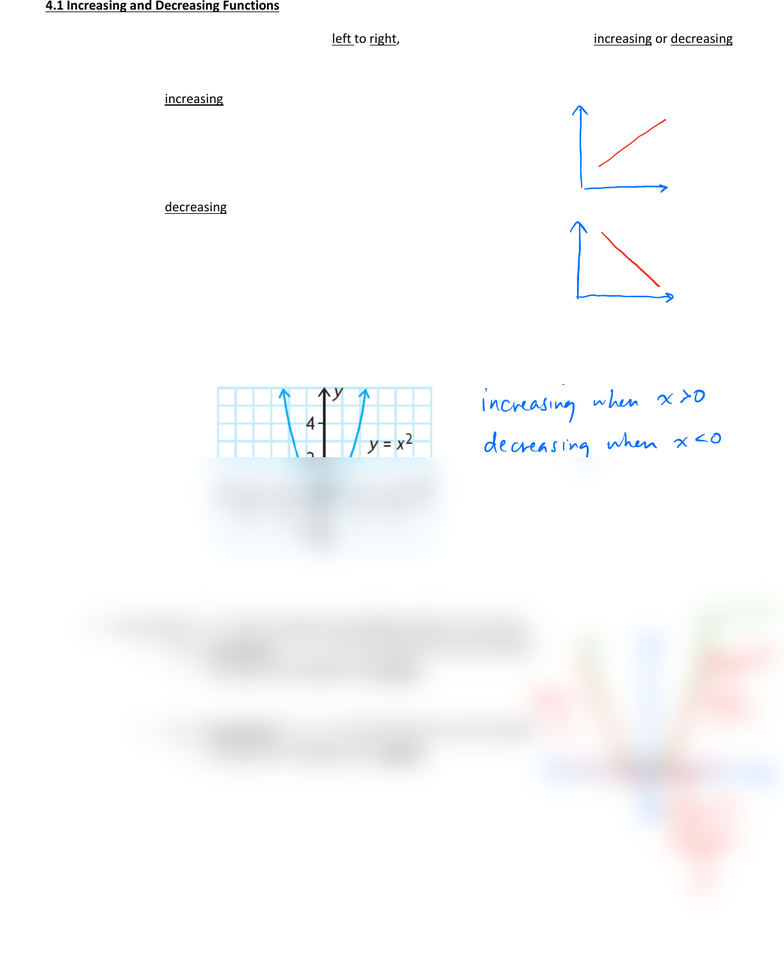# MAT135H1 Lecture 1: 4.1 Increasing and Decreasing Functions

42 views2 pages4.1 Increasing and Decreasing Functions
By reading a graph of a function from left to right, we can determine if a function is increasing or decreasing
on an interval
()fx
is increasing (rises) on an interval if,
! for any value of
12
xx< on the interval ,
12
() ( )fx fx<
()fx is decreasing (falls) on an interval if,
! for any value of
12
xx< on the interval ,
12
() ()fx fx>
Example 1: Given
2
()fx x= , determine the intervals where ()fx is increasing and decreasing
for a function ()fx that is continuous and differentiable on an interval,
o ()fx is increasing if '( ) 0fx
>
for all values of x on the interval
! the slope of the tangents are positive
o ()fx is decreasing if '( ) 0fx
<
for all values of x on the interval
! the slope of the tangents are negative
increasing when X0
decreasing when xso
i
slopeof
tangent
is
Unlock document

This preview shows half of the first page of the document.
Unlock all 2 pages and 3 million more documents.